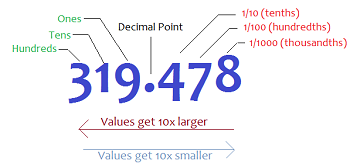## Math

Math has been going on in life since I was born. This subject is really important for us students to learn, but as a learner I know that math is really a complicated subject for almost every students. Even though it’s a grade one or a university math problems, but it still a difficult work until you learn, understand and practice it everyday and most of the time in your life and those problems are counting number, adding or subtracting different number. Everyday in your life, you spend money so you at least know how to count it, calculate it and also know the place of the number until a million place. Now, I do learn different kind of math, but for this term we are focusing on the percentage and angles. As you know, there are many things, living organism or even the economy in the country use percentage to tell the data or statistic. There are billion of animals so what we do is estimate it and calculate it as a percentage that is easier to memorize. Also, angles do take part in every of your daily life and connect a lot to physic. Even when you want to make a table, you need to talk about angle in it. So keep calm and learn math creatively as the way you understand it.

## DecimalsIn math class, we learn a different unit of math every day so for round 2 we learned about decimal. The beginning of class, it always the start of how you need to know and calculate it. For decimal, we start learning about the place of the decimal which the decimal start with tenth and continue to hundredth, thousandth and more. Calculate decimal is not difficult because it’s like calculate the whole number, but it weird when you multiply or divide with it. There are always tricks for math so is multiply and divide decimal. For multiplication, you need to move point to the left and for division move point to the right. The zero is always useless after the decimal. We also do Khan Academy to improve our decimal skill. For this term, it’s ended for decimal and continue to the next one.

## Geometry and Ratio

This is the second term of 2018 – 2019. We finished Primary Mathematics 5A book and about to start the new one. For this term, we are learning Geometry and Ratio a lot which give the ideas what it’s like to measure shapes and using ratio as a daily life in the future. For example, if you having a job and you need to manage your money on needs, wants and save which ratio would help. It’s also help comparing the different between things easily especially, gender.Example of having job and need to be decide in other needs, wants and save

For geometry, we are focusing a lot on finding areas especially for triangles and rectangles. As we start learning geometry, it’s leaning from first by counting grid and later calculation until solving harder and harder problems. Sometimes, it’s hard as it more difficult for the word problems, but that’s things need to be challenge in math. However, solving problems always have technique to solve it easily especially try to understand it at the very first start.## MathematicsIn mathematics class, we are learning about different things, but in this term mostly we are learning about fraction. It’s one of the lesson from our math book called Primary Mathematics 5A. It’s easy to learn how to calculate different things, but it will be more easy if I practice time to time. Also, In math class, calculation is not a problem, but the hard things to do is word problem which is not my first language. Even though, it is hard for me, but I still love it because it’s challenging with make my brain work faster.